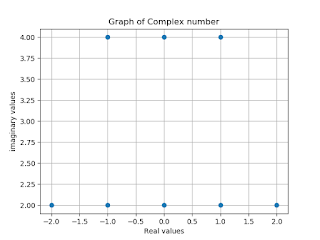## In this section, We discuss how to plot a complex number in Python and its operations. The various operations like Addition, Subtraction, Multiplication, Division and also discuss absolute and power functions. So move further first thing we know about what is a complex number and how it can be used in Python. Let's start with the introduction of a complex number.

Complex Numbers

Complex numbers are mostly used where we are using two real numbers. For example, an electrical circuit that is defined by voltage (V) and current (C) is used in geometry, scientific calculation, and calculus. A complex numbered object has two properties that are real (return the real component) and the imagery (excluding the imaginary component) the imaginary unit J).

Python complex()

A complex number is formed from real numbers. Python complex numbers can be created using the direct assignment statement or by using the complex () function.

syntax:

complex([real[, imag]])

Positive numbers always have two real roots. For example, if x2 is 25, then x is ±5. However, if x2 is -25 real roots are not present. The square root of any negative number is an imaginary unit j = √. Multiplying by 1 is the square root of its absolute value.

Therefore  √−25 = √25 𝑋−1 = √25 × √−1 = 5j
A complex number has real and imaginary components. It is denoted as x + yj. Both x and y are real numbers. Y multiplied by the imaginary unit forms an imaginary part of the complex number.

Examples: 3 + 2j, 10-5.5J, 9.55 + 2.3j, 5.11e-6 + 4j

Python complex numbers can be created using the complex () function as well as the direct assignment statement.

## Python cmath Module

The Python cmath module provides access to mathematical functions for complex numbers. This module is always available. Functions of this module accept integers, floating-point numbers, or complex numbers as integers.

They will also accept any Python object that has either a __complex __ () or a __float __ () method: these methods are used to transform a complex or floating-point number of objects, respectively, and the function is then applied to the resulting transform.

Now take a look at how we can work with complex numbers in Python.

`1. Python Code for Real Part`
```import numpy as np
x = (1+2j)
print(x.real)```
Output: 1.0

`2. Python Code for imaginary part`
```import numpy as np
x = (1+2j)
print(x.imag)```

Output:2.0

It also has the conjugate () method. The conjugation of a complex number has the same real component and imaginary component with the opposite sign.

Therefore the conjugation of 2 + 3j is 2-3j.
```import numpy as np
n = np.conjugate(2+3j)
print(n.conjugate())```
Output:(2-3j)

Python also has an underlying complex () function that returns a complex number object. The function takes two parameters, one for each real and imaginary component. It can be of any numeric type (int, float or complex).
`3. Python Code for Complex number`
```import numpy as np
x = np.complex(1,2)
print(x)
y = np.complex(3,4)
print(y)
```
Output:(1+2j)
`             (3+4j)`
If only one parameter is given then it is considered a real component, the imaginary component is considered zero.

The addition and subtraction of complex numbers are the same as an integer or float. Real and imaginary parts are added/subtracted separately.

4. Addition of two Complex numbers
```z = x+y
print(z)```
Output:(4+6j)

5. Subtraction of two Complex numbers
```a = x-y
print(a)```
Output:(-2-2j)

For multiplication, consider complex numbers as binomials and multiply each term in the second number by the first number.

6. Multiplication of two Complex numbers
```b = x*y
print(b)```
Output:(-5+10j)

7. Division of two Complex numbers
```c = x/y
print(c)
```
```Output:(0.44+0.08j)
```
8. Absolute function
```import numpy as np
p = 3+4jq = np.abs(p)
print(q)
```
```Output:5.0
```
9. Power function
```import numpy as np
s = 1+2jl = np.power(s,2)
print(l)```
Output:(-3+4j)

## Graphical Representation of Complex number

```import numpy as np
from matplotlib import pyplot as plt
l =([-2+2j,-1+2j,0+2j,1+2j,2+2j,-1+4j,0+4j,1+4j])
x = [x.real for x in l]
y = [y.imag for y in l]
plt.plot(x,y,'o')
plt.xlabel('Real values')
plt.ylabel('imaginary values')
plt.title('Graph of Complex number')
plt.grid(True)
plt.show()```
Output:To Plot Complex Number in Python